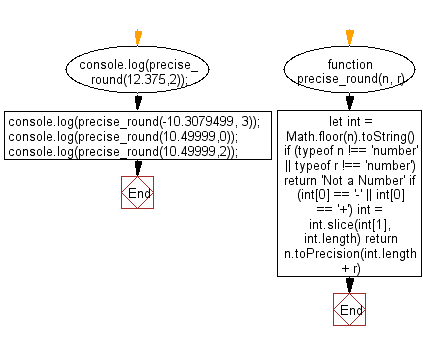# JavaScript: Round a number to a given specific decimal places

## JavaScript Math: Exercise-14 with Solution

Write a JavaScript function to round a number to decimal place.

Test Data:
console.log(precise_round(12.375,2));
console.log(precise_round(12.37499,2));
console.log(precise_round(-10.3079499, 3));
Output :
"12.38"
"12.37"
"-10.308"

Pictorial Presentation:Sample Solution:

HTML Code:

``````<!DOCTYPE html>
<html>
<meta charset="utf-8">
<title>Round a number to a given decimal places</title>
<body>
</body>
</html>
```
```

JavaScript Code:

``````//Ref.https://bit.ly/3zxAhnH
function precise_round(n, r) {
let int = Math.floor(n).toString()
if (typeof n !== 'number' || typeof r !== 'number') return 'Not a Number'
if (int == '-' || int == '+') int = int.slice(int, int.length)
return n.toPrecision(int.length + r)
}
console.log(precise_round(12.375,2));
console.log(precise_round(-10.3079499, 3));
console.log(precise_round(10.49999,0));
console.log(precise_round(10.49999,2));
```
```

Sample Output:

```12.38
-10.308
10
10.50
```

Flowchart:Live Demo :

See the Pen javascript-math-exercise-14 by w3resource (@w3resource) on CodePen.

Improve this sample solution and post your code through Disqus

What is the difficulty level of this exercise?

Test your Programming skills with w3resource's quiz.

﻿